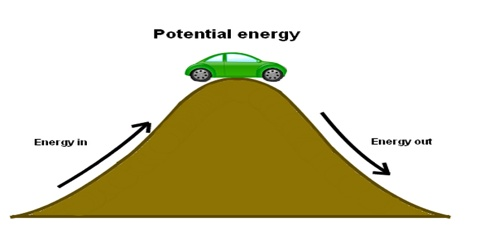# Experiment: Determination of Potential Energy of a Spring

Name of the experiment: Determination of potential energy of a spring

Theory: Let a weight of mass in be hanged at one end of a spring or the spring is expanded by an amount x due to application of force F. This displacement of the sprint is proportional to the applied force, i.e., F ∞ x

or, F = Kx [here, K = spring constant] … … … (1)

Now, work done by external force to expand the spring from position x1 to x2 is,

W = x2x1 F dx

= x2x1 Kx dx = K x2x1 x dx

= K [x2/2]x2x1 = K/2 (x22 – x12)

So, W = ½ K (x22 – x12) … … … (2)

This work is positive work. This work remains in the spring as potential energy.

Let, x1 = 0 and x2 = x so,

W = ½ K (x2 – 0)

or, W = ½ K x2

If a spring of mass m expands by ‘l’ and in this position if the spring is pulled by an amount x and released, then it executes simple harmonic motions. Its time period becomes, T = 2π √(m/K)Apparatus:

(1) An experimental spring

(2) One metre scale

(3) Some suitable weights

(4) A hook to hang the spring

(5) A stop watch

Procedure

(1) The spring is to be hanged as per figure.

(2) At one end of the spring if a weight is hanged than the spring will be slightly elongated. Now unsure the distance between the stationary position and the changed position of the spring by a metre scale. This is gives the elongated length ‘l’.

(3) Then the weight is pulled downward by a distance x and released. Again, the expansion of length x is measured by the metre scale.

(4) The spring will continue moving ups and down. Time is recorded by a stop watch for 20 complete oscillations. Dividing this time by 20 time period T is to be measured.

(5) By changing weights the processes (3) and (4) are repeated for a few times.

Data Table- Table for the determination of T and xCalculations: l = … … m, and x = … … m

Time period, T = 2π√(m/K)

So, K = ……

Potential energy, W = ½ Kx2 = … … Joule

Precautions and discussion:

(1) The spring is to be hanged in such a way so that it does not come out from the hook after hanging the load

(2) Extension of the spring is to be increased slowly in steps

(3) Care should be taken so that no hindrance occurs during expansion of spring due to hanging of weight at the end of the spring

(4) Cathodometer should be used for measuring accurate length.